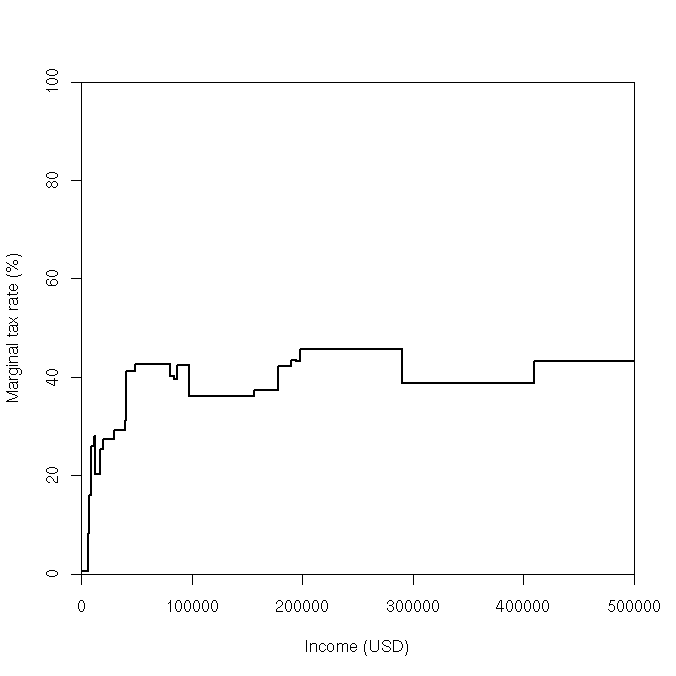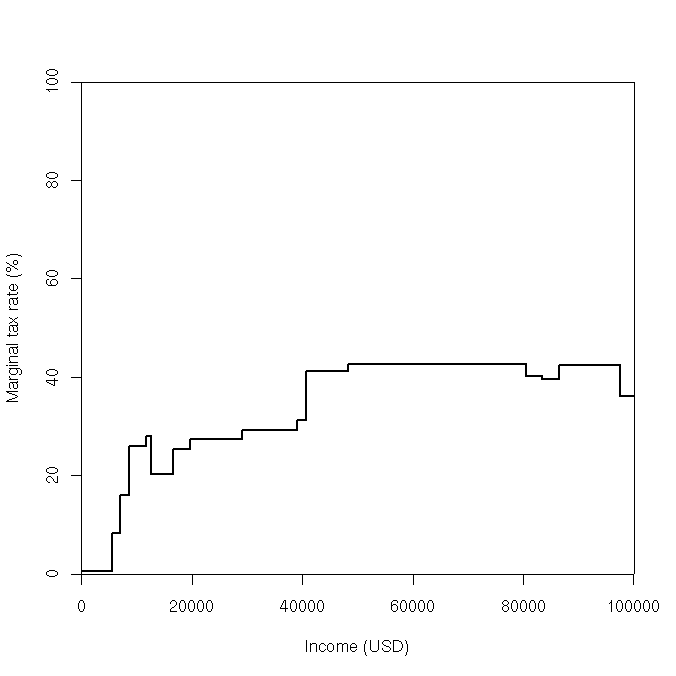# Marginal income tax rates for a single California resident with only wage income, 2007

As I did some taxes this year (March 2008), going through some of the forms (by hand, as I always do, partly since I like to understand what it is that I'm paying) made me realize how bizarre our tax system is.

Consider the following relatively simple example: a single (non-dependent) person in California earning all of their income from wages, salaries, and tips. (Once antipoverty programs like the Earned Income Credit aren't relevant, this assumption could be relaxed to allow income from many other sources as well, although capital gains are taxed differently.) Assume further that this person's withholding is perfect so that they can deduct exactly their 2007 state taxes from their federal returns once they get to the point of itemizing deductions (rather than having some variation from year to year due to imperfect withholding). Consider the effect of the following taxes:

US Income Tax
progressive rate with some initial income excluded due to subtracting standard deduction and exemption amounts, with deductions itemized once it becomes worth itemizing deductions (rather than taking the standard deduction) based on state taxes paid alone (see two California taxes below)
Social Security Tax
6.2% of income under \$97500 (ignoring that it's really double that because the employer pays the same)
Medicare Tax
1.45% of income (ignoring that it's really double that because the employer pays the same)
US Alternative Minimum Tax
A separate progressive tax structure that allows fewer deductions, forcing rich people to give up some of their itemized deductions if this structure produces a larger tax than the normal tax structure.
California Income Tax
progressive rate with some initial income excluded due to subtracting the standard deduction and exemption amounts
California State Unemployment/Disability Insurance
0.6% of income under \$83388.33
Earned Income Credit
A federal antipoverty program that subtracts from income tax (potentially making it negative). Doesn't pay much to people without children, so doesn't figure much here.

This yields the marginal rates shown in the following graph:And here's a zoom of the part of the graph under \$100,000:One way to understand what this graph represents is that if a person meeting these conditions drew a vertical line at their income, the area to the left of that line would be everything they earned. The part below the line drawn would be the part paid in taxes, and the part above would be the part they keep.

[ Edit, May 12, 2010 -- The reason I called these graphs bizarre isn't the magnitude of the numbers; they're in the range one would expect for funding a modern government. What's bizarre about these graphs is their shape. There is no good reason for this graph to have lots of bumps in it. I'd expect a line that's gradually increasing (perhaps stepwise), not one that goes up and down a lot. I think the bumps are a result of the bad process we use for making policy. -- end Edit]

The details of these marginal rates are:

1. Thanks to standard deductions and dependent exemptions (since the taxpayer has himself as a dependent), both federal and California income taxes don't kick in immediately. However, the Earned Income credit does (at a rate of -7.65%), so the initial marginal rate is 0.6% when subtracting that credit from the other three taxes. (This ignores other antipoverty programs, though.)
2. Around \$5550 (where the total tax paid is around \$33.30), the Earned Income Credit changes to a flat \$428, bringing the marginal rate up to the rate of Social Security plus Medicare plus California SUI/SDI, which is 8.25%.
3. Around \$7000 (where the total tax is around \$150), the Earned Income Credit starts decreasing. (This is for single people; for people with children it's still increasing (not even flat yet) at this income level.) This brings the marginal rate up to 15.9%.
4. At \$8750 (where the total tax is around \$428), we've used up the federal standard deduction and 1 exemption, so federal income taxes kick in at 10%, making the marginal rate 25.9%
5. At \$11629 (where the total tax is around \$1174), we've used up California's standard deduction (\$3516) and the \$94 after-tax exemption for supporting oneself, so California income taxes kick in at a marginal rate of 2%, making the total marginal rate 27.9%.
6. At \$12590 (where the total tax is around \$1442), the Earned Income Credit has hit 0, so the total marginal rate drops to 20.25%.
7. At \$16575 (where the total tax is around \$2249), the federal income tax rate goes up to 15%, so the total marginal rate is 25.25%
8. At \$19701 (where the total tax is around \$3038), the California income tax rate goes up to 4%, so the total marginal rate is 27.25%
9. At \$29060 (where the total tax is around \$5588), the California income tax rate goes up to 6%, so the total marginal rate is 29.25%
10. At \$38976 (where the total tax is around \$8489), the California income tax rate goes up to 8%, so the total marginal rate is 31.25%
11. At \$40600 (where the total tax is around \$8996), the Federal income tax rate goes up to 25%, so the total marginal rate is 41.25%
12. At \$48330 (where the total tax is around \$12185), the California income tax rate goes up to 9.3% (the top bracket), so the total marginal rate is 42.55%
13. At \$80460.81 (where the total tax is around \$25857), total payments of income taxes to California now equal the federal standard deduction (\$5350), so it becomes worth itemizing deductions on federal taxes. The marginal rate for total California taxes is 9.9%. This means that 9.9% of each additional dollar doesn't count towards federal income tax, reducing its effective marginal rate from 25% to 22.525%, making the total marginal rate 40.075%.
14. At \$83388.33 (where the total tax is around \$27030), the maximum payment into California state disability and unemployment insurance (\$500.33) is paid. This, in turn, reduces the total marginal rate of state taxes to 9.3%, which increases the effective federal income tax marginal rate (remember the last step) to 22.675%, making the total marginal rate 39.625%
15. Around \$86421.96 (where the total tax is around \$28232), the Federal income tax rate goes up to 28%. Remember we're deducting 9.3% of income, though, so the total marginal rate is 42.346%
16. At \$97500 (where the total tax is around \$32923), Social Security taxes stop, so the total marginal rate is 36.146%
17. At \$156400 (where the total tax is around \$54215), Federal exemption and deduction limiting kicks in, the former in a series of discrete jumps of \$12.69 each (1.181333% slope), and the latter continuously (at a 7.3% slope). Around the same point (actually the first step is at \$156666, but treating it as a continuous function it starts at \$155416), California exemptions start being limited as well. Since this is a very small effect: a series of \$4.32 steps for every increment of \$2500 in income (\$6 more in California tax, minus the offset that causes in Federal tax), I'll just throw it in here as well. Treating all of these as continuous, this makes the total marginal rate around this point change to approximately 37.39%.
18. At an income of \$177914.32 (where the total tax is around \$62258), the Federal tax rate goes up to 33%, changing the total marginal rate to approximately 42.1%.
19. At an income of \$190153.08 (where the total tax is around \$67411), the alternative minimum tax kicks in (with the alternative structure passing the regular income tax structure), and the total marginal rate changes to 43.49%. Note that even with small amounts of income from capital gains, this can happen at significantly lower income, because the alternative minimum tax's steep exemption phaseout does include capital gains (whereas almost nothing else does).
20. Around an income of \$194582.66 (assuming a continuous function, which it really isn't), the California exemption hits zero (so the gradual limiting stops), and the marginal rate drops to 43.25%.
21. At \$197980 (where the total tax is around \$70807), the alternative minimum tax rate jumps from 26% to 28% (though the effective rate is larger, jumping here from 32.5% to 35%, since the AMT exemption limiting is still being phased in), making the total marginal rate now 45.75%.
22. At \$289900 (where the total tax is around \$112860), the AMT exemption is now limited down to zero, which makes the federal income tax marginal rate drop to the AMT's official 28%, making the total marginal rate now 38.75%.
23. At \$409665.88 (where the total tax is around \$159269), the regular federal income tax rate schedule (with its now higher marginal rates) crosses above the alternative minimum tax again, making the total marginal rate now 43.195%. This is the marginal rate on income from this point up (given these very simple assumptions).

I probably made a whole bunch of mistakes here, never mind the major pieces I omitted, but this seesaw graph starts to paint a picture of the US tax system. It has so many details and patches to fix little things that the big picture is a mess.

I think I'd probably prefer a graduated (and actually progressive) tax on consumption (allowing major purchases like houses and cars to be treated as investments, consuming only the fair market value of the opportunity cost of not renting it). This could be done through a mechanism similar to the one we have now (but simpler), except where savings are deductable and loans are taxable.

See the Haskell program that I used to figure a bunch of this out, as well as the data and R code that I used for the plot.

David Baron, dbaron@dbaron.org, 2008-03-17